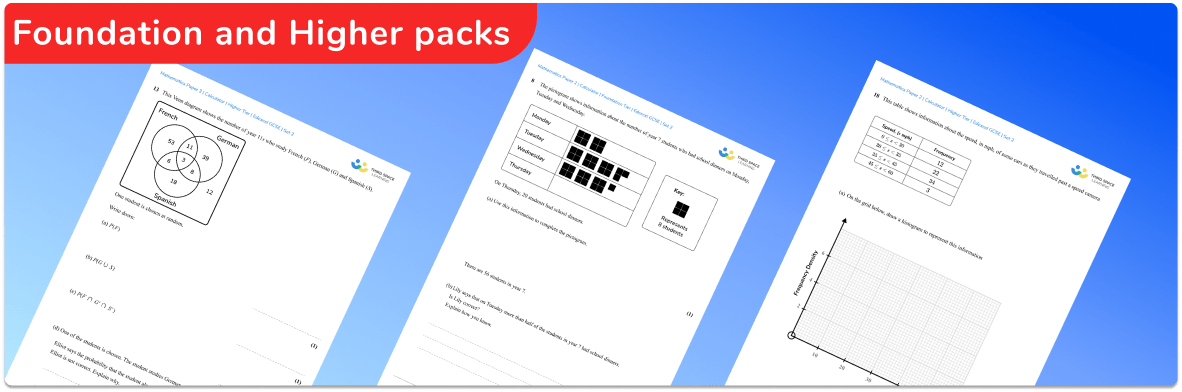# Practice Paper Pack: Edexcel Foundation and Higher 2023 Set 2This practice paper pack for students following the Edexcel exam board, contains 3 foundation practice papers (1 calculator, 2 non-calculator) and 3 higher practice papers (1 calculator, 2 non-calculator)

• Unique and original questions are closely matched to the style and format of Edexcel past GCSE maths papers
• Answers and detailed mark scheme provided
• All Edexcel maths paper questions are created by current or former examiners and expert maths teachers
• Includes a practice paper index which shows how the question numbers in the example papers relate to the specific topics